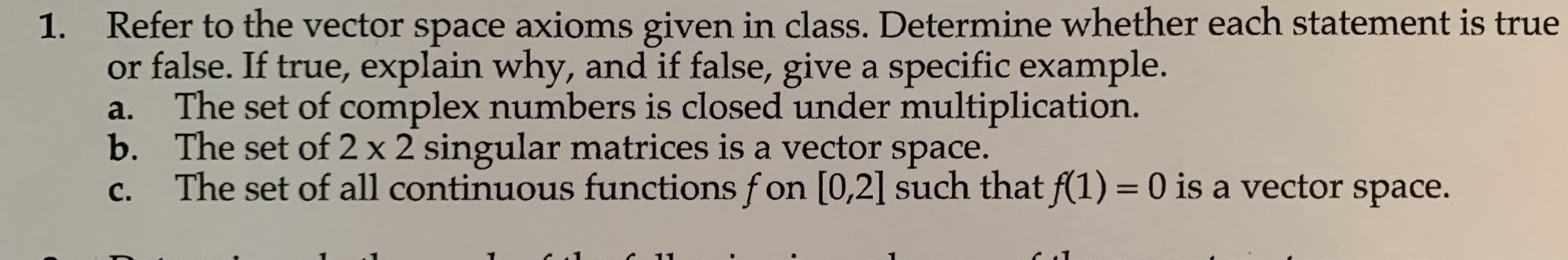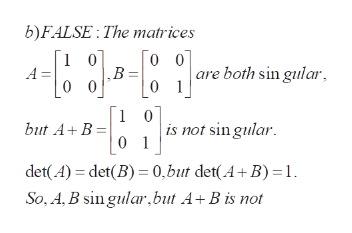# Refer to the vector space axioms given in class. Determine whether each statement is trueor false. If true, explain why, and if false, give a specific example.The set of complex numbers is closed under multiplication.b. The set of 2 x 2 singular matrices is a vector space.The set of all continuous functions fon [0,2] such that f(1) 0 is a vector space.1.a.с.

Question
1 viewshelp_outlineImage TranscriptioncloseRefer to the vector space axioms given in class. Determine whether each statement is true or false. If true, explain why, and if false, give a specific example. The set of complex numbers is closed under multiplication. b. The set of 2 x 2 singular matrices is a vector space. The set of all continuous functions fon [0,2] such that f(1) 0 is a vector space. 1. a. с. fullscreen
check_circle

Step 1

To find the truth or falsity of the given statements

Step 2

a) LTRUE: the product of any two complex numbers a+ib and c+id is again a complex number.

Step 3

b)FALSE: By definition, a square matrix is singular if its determinant is 0. The example shown proves th...help_outlineImage Transcriptioncloseb)FALSE: The matrices 1 0 0 0 are both sin gular A ,В. 0 0 0 1 1 0 is not sin gular 0 1 but A+ B det(A) det(B) 0,but det(A+ B) 1 So, A,B sin gular,but A+B is not fullscreen

### Want to see the full answer?

See Solution

#### Want to see this answer and more?

Solutions are written by subject experts who are available 24/7. Questions are typically answered within 1 hour.*

See Solution
*Response times may vary by subject and question.
Tagged in

### Math